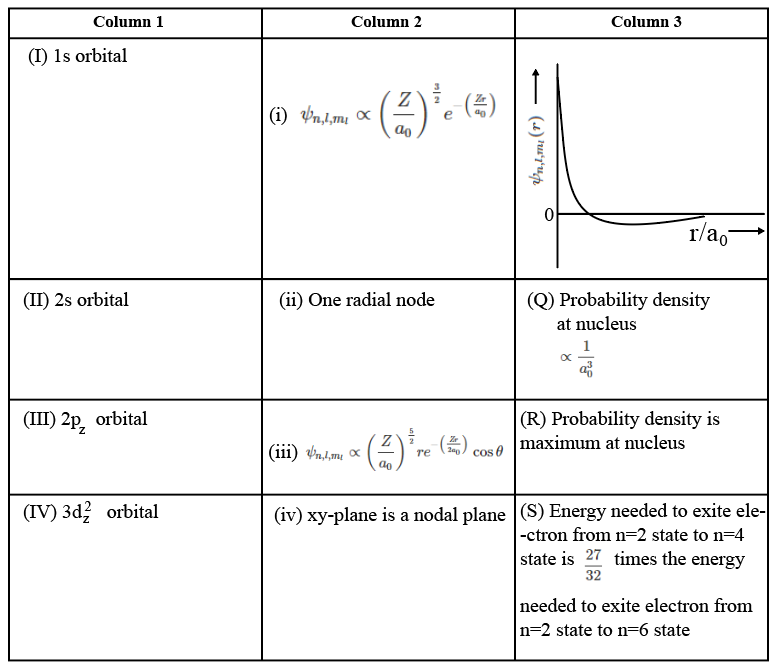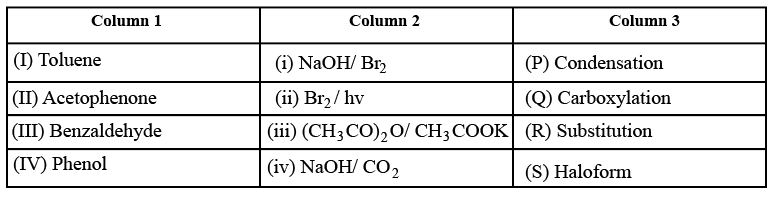Instructions

Answer the Questions by appropriately matching the information given in the three columns of the following table.

The wave function, $$\psi_{n, l, m_1}$$ is a mathematical function whose value depends upon spherical polar coordinates $$(r, \theta, \phi)$$ of the electron and characterized by the quantum numbers n, l and $$m_1$$. Here r is distance from nucleus, $$\theta$$ is colatitude and $$\phi$$ is azimuth. In the mathematical function given in the Table, Z is atomic number and $$a_0$$ is bohr radius.Question 31

Question 32

Question 33

# For $$He^+$$ ion, the only INCORRECT combination is

Instructions

Answer the Questions by appropriately matching the information given in the three columns of the following table.

Columns 1, 2 and 3 contain starting materials, reaction conditions, and type of reactions, respectively.Question 34

Question 35

Question 36

# The only CORRECT combination in which the reaction proceeds through radical mechanism is

Instructions

For the following questions answer them individually

Question 37

Question 38

Question 39

Question 40

OR